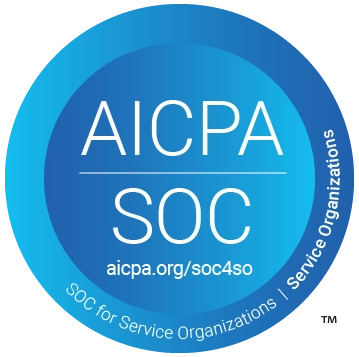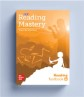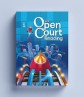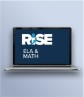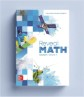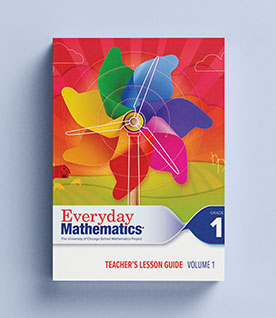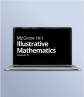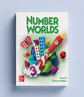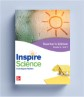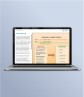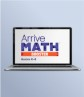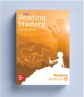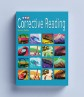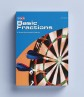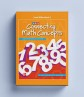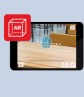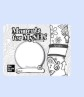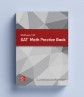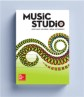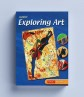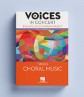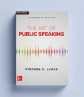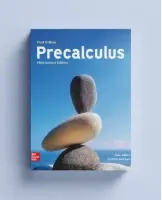## Our Principles# Pre-Algebra with Pizzazz!

Pre-Algebra with Pizzazz! features intriguing riddles, jokes and limericks that provide pre-algebra concepts and skills practice. Reproducible activity pages contain self-checking solutions and are organized by topic for easy use.

## Program Details

Features:

• Section AA - Integers, fractions, decimals, and properties of operations
• Section BB - Exponents, decimals, scientific notation, irrational numbers, ratio, proportion, percent and probability
• Section CC - Geometric figures, measurement, square roots, Pythagorean Theorem, trigonometric ratios, and statistics
• Section DD - Variables and expressions, equations, problem solving, inequalities, functions, and graphing
##### Get Help